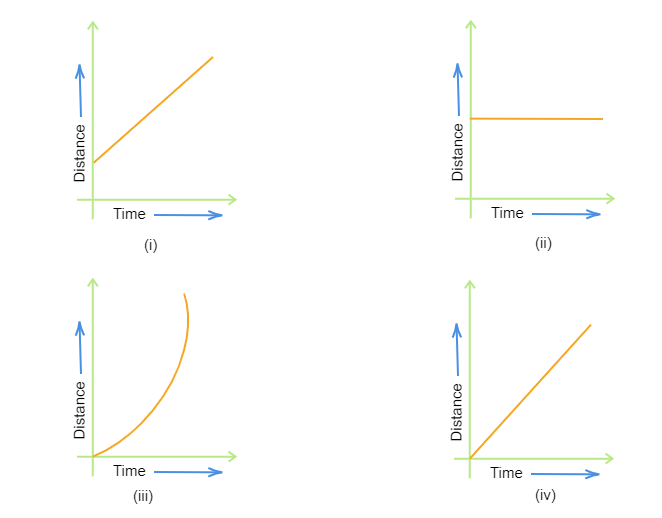" ">

# Which of the following distance-time graphs shows a truck moving with a speed which is not constant?"

Given:

Four distance-time are given in the question above.

To do:

To determine the distance-time graph that shows a truck moving with a speed that is not constant.

Solution:

The slope of a distance-time graph represents the motion of the object. let us observe the slopes of the given distance-time graphs and find the graph that shows a truck moving with a speed which is not constant.

Observations of slopes of the given graphs:

Graph $(i)$

In the given graph slope is a straight line representing uniform motion. It represents the constant speed.

Graph $(ii)$

The distance covered is not increasing with increasing time in graph $(ii)$.

So, it represents that the object is at rest.

Graph $(iii)$

The slope of Graph $(iii)$ is curved. So, it does not represent the constant speed of the object. It represents the variable speed as its slope is curved.

Graph $(iv)$
The slope of graph $(iv)$ is a straight line that represents the constant speed of the object.

Conclusion:

From the observations of the slopes of the given graphs, it is clear that graph $(iii)$ is the only graph that shows a truck moving with a speed that is not constant.

Updated on: 10-Oct-2022

77 Views